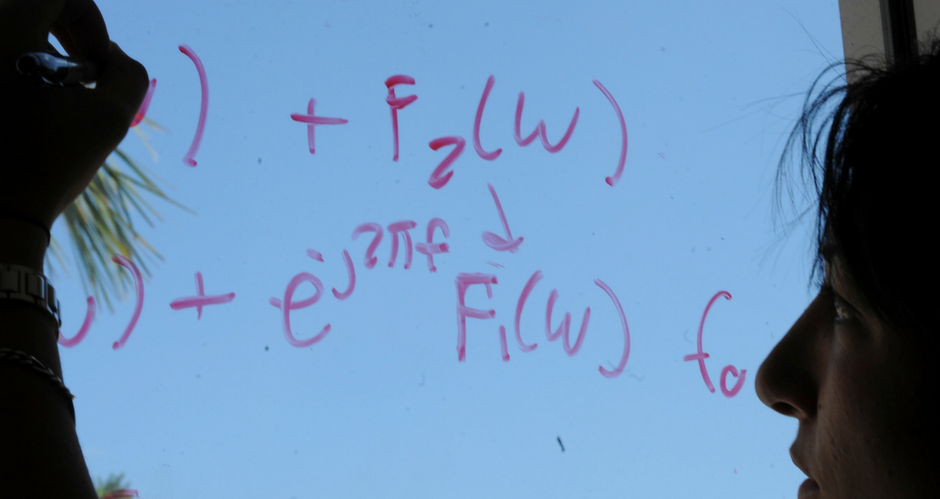## Mathematics and System Engineering Faculty Publications

### Oscillation Criteria for Second-Order Forced Dynamic Equations with Mixed Nonlinearities

Article

#### Abstract

We obtain new oscillation criteria for second-order forced dynamic equations on time scales containing mixed nonlinearities of the form (r(t) Φα (xΔ))Δ + f (t, xσ) = e(t), t ∈ [t0, ∞) T with f(t, x) = q(t)Φα(x) + ∑i=1nqi(t)Φβi (x), Φ* (u) = u *-1u, where [t0, ∞)T is a time scale interval with t0 ∈ T, the functions r, q, qi, e: [t0, ∞)T → ℝ are right-dense continuous with r > 0, σ is the forward jump operator, xσ(t) := x (σ(t)), and β1 > ⋯ > βm > α > βm+1 > ⋯ βn > 0. All results obtained are new even for T = ℝ and T = ℤ. In the special case when T = ℝ and α = 1 our theorems reduce to (Y. G. Sun and J. S. W. Wong, Journal of Mathematical Analysis and Applications. 337 (2007), 549-560). Therefore, our results in particular extend most of the related existing literature from the continuous case to arbitrary time scale.

#### DOI

10.1155/2009/938706

6-2-2009

COinS